Department of Pre-University Education, Karnataka course PUC Karnataka Science Class 12
Share

# Take C 1 = 4.0 Uf and C 2 = 6.0 Uf in Figure . Calculate the Equivalent Capacitance of the Combination Between the Points Indicated. - Physics

ConceptCapacitors and Capacitance

#### Question

Take C_1 = 4.0  "uF" and C_2 = 6.0  "uF" in figure . Calculate the equivalent capacitance of the combination between the points indicated.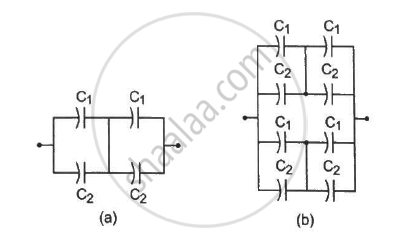#### Solution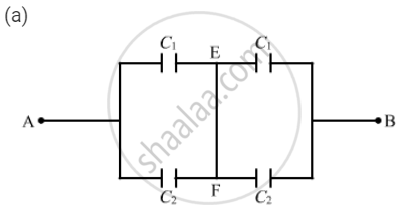For the combination of capacitors given in figure (a), the pairs of capacitors C1 and C2are in parallel.
The equivalent capacitance of each parallel combination of capacitors is given by
C1 C2 = 4 + 6 = 10 μF
The equivalent circuit can be drawn as :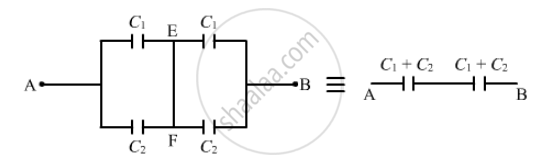The  equivalent capacitance for the above series circuit is given by

1/C_(eq) = 1/(C_1 + C_2) + 1/(C_1+C_2) = 1/10 + 1/10 = 2/10

⇒ C_("eq") = 5  "uF"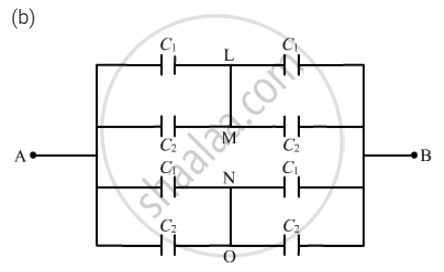For the combination of capacitors given in figure (b), the pairs of capacitors C1 and C2are in parallel.
The equivalent capacitance of each parallel combination of capacitors is given by
C1 C2 = 4 + 6 = 10 μF

The equivalent circuit can be drawn as :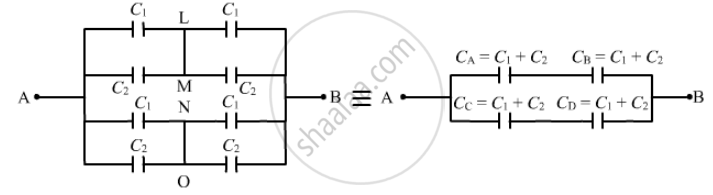In the above circuit, it can be seen that Cand CB are in series and are in parallel to the series combination of Cand CB.

The equivalent capacitance for the series combination of Cand Cis given by

1/C_(eq) = 1/C_A + 1/C_B = 1/(C_1+C_2) + 1/(C_1 + C_2)= 1/10 + 1/10 = 1/5

⇒ C_(eq) = 5  "uF"

Similarly, the equivalent capacitance of the series combination of Cand Cis 5 μF.

∴ Net equivalent capacitance = 5 + 5 = 10 μF

Is there an error in this question or solution?

#### Video TutorialsVIEW ALL 

Solution Take C 1 = 4.0 Uf and C 2 = 6.0 Uf in Figure . Calculate the Equivalent Capacitance of the Combination Between the Points Indicated. Concept: Capacitors and Capacitance.
S